# TensorFlow从1到2（十）#### 基本概念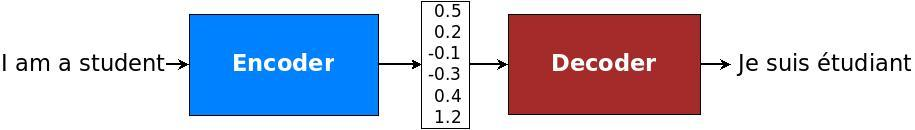（图片来自谷歌NMT文档）

（图片来自互联网）

``````	C = Encoder("I love you")
``````

``````	"我" = Decoder(C)
"爱" = Decoder(C)
"你" = Decoder(C)
``````

``````	"我" = Decoder(C+"I")
"爱" = Decoder(C+"love")
"你" = Decoder(C+"you")
``````

``````	"我" = Decoder(C+0.8x"I"+0.1x"love"+0.2x"you")
"爱" = Decoder(C+0.1x"I"+0.7x"love"+0.1x"you")
"你" = Decoder(C+0.2x"I"+0.1x"love"+0.8x"you")
``````

#### 翻译模型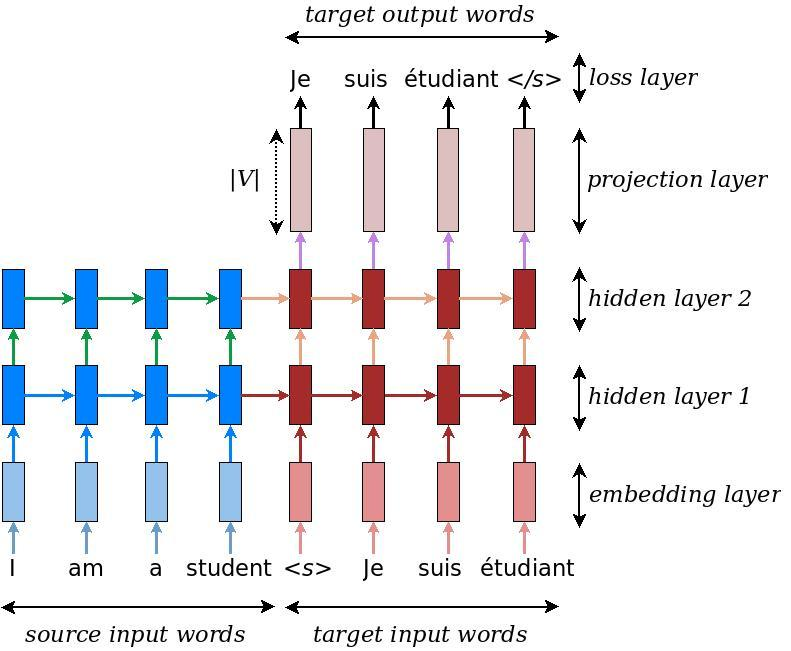（图片来自谷歌NMT文档）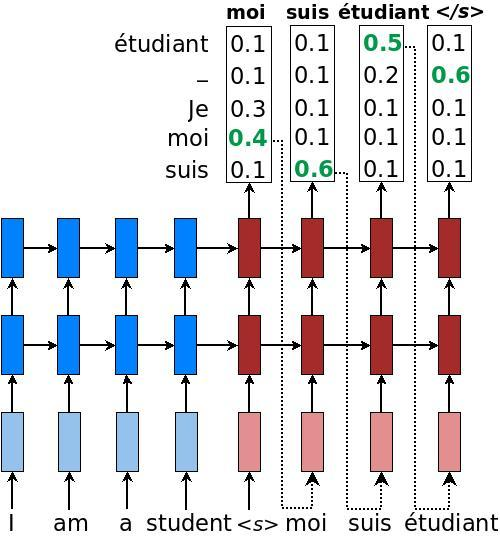（图片来自谷歌NMT文档）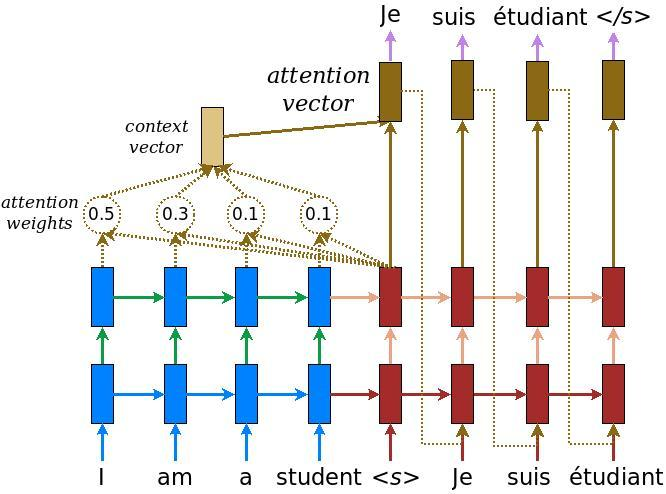（图片来自谷歌NMT文档）

#### 样本及样本预处理

``````May I borrow this book? ¿Puedo tomar prestado este libro?
``````

• 读取样本库，建立数据集。每一行的样本按语言分为两个部分。
• 为每一句样本，增加开始标志`<start>`和结束标志`<end>`。看过《从锅炉工到AI专家(10)》的话，你应当理解这种做法。经过训练后，模型会根据这两个标志作为翻译的开始和结束。

``````<start> may i borrow this book ? <end>
<start> ¿ puedo tomar prestado este libro ? <end>
``````

• 进行数据清洗，去掉不支持的字符。
• 把单词数字化，建立从单词到数字和从数字到单词的对照表。
• 设置一个句子的最大长度，把每个句子按照最大长度在句子的后端补齐。

``````Input Language; index to word mapping
1 ----> <start>
8 ----> no
38 ----> puedo
804 ----> confiar
20 ----> en
1000 ----> vosotras
3 ----> .
2 ----> <end>

Target Language; index to word mapping
1 ----> <start>
4 ----> i
25 ----> can
12 ----> t
345 ----> trust
6 ----> you
3 ----> .
2 ----> <end>
``````

#### 模型构建

• 继承自keras.Model类。
• 实现`__init__`方法，用于实现类的初始化，同所有面向对象的语言一样，这里主要完成基类和类成员的初始化工作。
• 实现call方法，这是主要的计算逻辑。模型接入到神经网络之后，训练逻辑和预测逻辑，都通过逐层调用call方法来完成计算。方法中可以使用keras中原有的网络模型和自己的计算通过组合来完成工作。

Encoder/Decoder主体都使用GRU网络，读起来应当比较容易理解。有需要的话，复习一下《从锅炉工到AI专家(10)》

##### 训练和预测

• 编码器Encoder是一次整句编码，得到一个enc_output。enc_output相当于模型对整句语义的理解。
• 解码器Decoder是逐个单词输入，逐个单词输出的。训练时，输入序列由`<start>`起始标志开始，到`<end>`标志结束。预测时，没有人知道这一句翻译的结果是多少个单词，就是逐个获取Decoder的输出，直到得到一个`<end>`标志。
• Encoder和Decoder都引出了隐藏层，用于计算注意力权重。keras.layers.GRU的state输出其实就是隐藏层，平时这个参数我们是用不到的。
• 对于每一个翻译的输出词，注意力对其影响就是通过`attention_weights * values`，然后将结果跟前一个输出词一起作为Decoder的GRU输入，values实际就是编码器输出enc_output。
• Decoder输出上一个词时候的隐藏层，跟enc_output一起通过公式计算，得到下一个词的注意力权重attention_weights。在第一次循环的时候Decoder还没有输出过隐藏层，这时候使用的是Encoder的隐藏层。
• 注意力权重attention_weights从程序逻辑上并不需要引出，程序中在Decoder中输出这个值是为了绘制注意力映射图，帮助你更好的理解注意力机制。所以如果是在这个基础上做翻译系统，输出权重值到模型外部是不需要的。
• 为了匹配各个网络的不同维度和不同形状，注意力机制的计算逻辑和注意力权重经过了各种维度变形。Decoder的输入虽然是一个词，但也需要扩展成一批词的第一个元素（也是唯一一个元素），这个跟我们以前的模型在预测时所做的是完全一样的。

#### 完整源码

``````#!/usr/bin/env python3

from __future__ import absolute_import, division, print_function, unicode_literals

import tensorflow as tf

import matplotlib.pyplot as plt
from sklearn.model_selection import train_test_split

import unicodedata
import re
import numpy as np
import os
import io
import time
import sys

# 如果命令行增加了参数'train'则进入训练模式，否则按照翻译模式执行
TRAIN = False
if len(sys.argv) == 2 and sys.argv == 'train':
TRAIN = True

# 下载样本集，下载后自动解压。数据保存在路径：~/.keras/datasets/
path_to_zip = tf.keras.utils.get_file(
'spa-eng.zip',
extract=True)
# 指向解压后的样本文件
path_to_file = os.path.dirname(path_to_zip)+"/spa-eng/spa.txt"

# 将文本从unicode编码转换为ascii编码
def unicode_to_ascii(s):
return ''.join(
c for c in unicodedata.normalize('NFD', s)
if unicodedata.category(c) != 'Mn')

# 对所有的句子做预处理
def preprocess_sentence(w):
w = unicode_to_ascii(w.lower().strip())

# 在单词和标点之间增加空格
# 比如: "he is a boy." => "he is a boy ."
w = re.sub(r"([?.!,¿])", r" \1 ", w)
w = re.sub(r'[" "]+', " ", w)

# 用空格替换掉除了大小写字母和"."/ "?"/ "!"/ ","之外的字符
w = re.sub(r"[^a-zA-Z?.!,¿]+", " ", w)
# 截断两端的空白
w = w.rstrip().strip()

# 在句子两端增加开始和结束标志
# 这样经过训练后，模型知道什么时候开始和什么时候结束
w = '<start> ' + w + ' <end>'
return w

# 载入样本集，对句子进行预处理
# 最终返回(英文,西班牙文)这样的配对元组
def create_dataset(path, num_examples):

word_pairs = [[preprocess_sentence(w) for w in l.split('\t')]  for l in lines[:num_examples]]

return zip(*word_pairs)
# 至此的输出为：
# <start> go away ! <end>
# <start> salga de aqui ! <end>
# 这样的形式。

# 获取最长的句子长度
def max_length(tensor):
return max(len(t) for t in tensor)

# 将单词数字化之后的数字<->单词双向对照表
def tokenize(lang):
lang_tokenizer = tf.keras.preprocessing.text.Tokenizer(
filters='')
lang_tokenizer.fit_on_texts(lang)

tensor = lang_tokenizer.texts_to_sequences(lang)

tensor,

return tensor, lang_tokenizer

# 载入样本，两种语言分别保存到两个数组
targ_lang, inp_lang = create_dataset(path, num_examples)
# 把句子数字化，两种语言是两套对照编码
input_tensor, inp_lang_tokenizer = tokenize(inp_lang)
target_tensor, targ_lang_tokenizer = tokenize(targ_lang)

return input_tensor, target_tensor, inp_lang_tokenizer, targ_lang_tokenizer

# 训练的样本集数量，越大翻译效果越好，但训练耗时越长
num_examples = 80000
input_tensor, target_tensor, inp_lang, targ_lang = load_dataset(path_to_file, num_examples)
# 至此，input_tensor/target_tensor 是数字化之后的样本（数字数组）
# inp_lang/targ_lang 是数字<->单词编码对照表
# 计算两种语言中最长句子的长度
max_length_targ, max_length_inp = max_length(target_tensor), max_length(input_tensor)

# 将样本按照8:2分为训练集和验证集
input_tensor_train, input_tensor_val, target_tensor_train, target_tensor_val = train_test_split(input_tensor, target_tensor, test_size=0.2)

##############################################

BUFFER_SIZE = len(input_tensor_train)
BATCH_SIZE = 64
steps_per_epoch = len(input_tensor_train)//BATCH_SIZE
embedding_dim = 256
units = 1024
vocab_inp_size = len(inp_lang.word_index)+1
vocab_tar_size = len(targ_lang.word_index)+1

dataset = tf.data.Dataset.from_tensor_slices((input_tensor_train, target_tensor_train)).shuffle(BUFFER_SIZE)
dataset = dataset.batch(BATCH_SIZE, drop_remainder=True)

# 编码器模型
class Encoder(tf.keras.Model):
def __init__(self, vocab_size, embedding_dim, enc_units, batch_sz):
super(Encoder, self).__init__()
self.batch_sz = batch_sz
self.enc_units = enc_units
self.embedding = tf.keras.layers.Embedding(vocab_size, embedding_dim)
self.gru = tf.keras.layers.GRU(
self.enc_units,
return_sequences=True,
return_state=True,
recurrent_initializer='glorot_uniform')

def call(self, x, hidden):
x = self.embedding(x)
output, state = self.gru(x, initial_state=hidden)
return output, state

def initialize_hidden_state(self):
return tf.zeros((self.batch_sz, self.enc_units))

encoder = Encoder(vocab_inp_size, embedding_dim, units, BATCH_SIZE)

# 注意力模型
class BahdanauAttention(tf.keras.Model):
def __init__(self, units):
super(BahdanauAttention, self).__init__()
self.W1 = tf.keras.layers.Dense(units)
self.W2 = tf.keras.layers.Dense(units)
self.V = tf.keras.layers.Dense(1)

def call(self, query, values):
# query为上次的GRU隐藏层
# values为编码器的编码结果enc_output
hidden_with_time_axis = tf.expand_dims(query, 1)

# 计算注意力权重值
score = self.V(tf.nn.tanh(
self.W1(values) + self.W2(hidden_with_time_axis)))

attention_weights = tf.nn.softmax(score, axis=1)

# 使用注意力权重*编码器输出作为返回值，将来会作为解码器的输入
context_vector = attention_weights * values
context_vector = tf.reduce_sum(context_vector, axis=1)

return context_vector, attention_weights

# 解码器模型
class Decoder(tf.keras.Model):
def __init__(self, vocab_size, embedding_dim, dec_units, batch_sz):
super(Decoder, self).__init__()
self.batch_sz = batch_sz
self.dec_units = dec_units
self.embedding = tf.keras.layers.Embedding(vocab_size, embedding_dim)
self.gru = tf.keras.layers.GRU(
self.dec_units,
return_sequences=True,
return_state=True,
recurrent_initializer='glorot_uniform')
self.fc = tf.keras.layers.Dense(vocab_size)

self.attention = BahdanauAttention(self.dec_units)

def call(self, x, hidden, enc_output):
# 使用上次的隐藏层（第一次使用编码器隐藏层）、编码器输出计算注意力权重
context_vector, attention_weights = self.attention(hidden, enc_output)

x = self.embedding(x)

# 将上一循环的预测结果跟注意力权重值结合在一起作为本次的GRU网络输入
x = tf.concat([tf.expand_dims(context_vector, 1), x], axis=-1)

# state实际是GRU的隐藏层
output, state = self.gru(x)

output = tf.reshape(output, (-1, output.shape))

x = self.fc(output)

return x, state, attention_weights

decoder = Decoder(vocab_tar_size, embedding_dim, units, BATCH_SIZE)

loss_object = tf.keras.losses.SparseCategoricalCrossentropy(from_logits=True)

# 损失函数
def loss_function(real, pred):
loss_ = loss_object(real, pred)

return tf.reduce_mean(loss_)

# 保存中间训练结果
checkpoint_dir = './training_checkpoints'
checkpoint_prefix = os.path.join(checkpoint_dir, "ckpt")
checkpoint = tf.train.Checkpoint(optimizer=optimizer,
encoder=encoder,
decoder=decoder)

# 一次训练
@tf.function
def train_step(inp, targ, enc_hidden):
loss = 0

# 输入源语言句子进行编码
enc_output, enc_hidden = encoder(inp, enc_hidden)
# 保留编码器隐藏层用于第一次的注意力权重计算
dec_hidden = enc_hidden

# 解码器第一次的输入必定是<start>，targ_lang.word_index['<start>']是转换为对应的数字编码
dec_input = tf.expand_dims([targ_lang.word_index['<start>']] * BATCH_SIZE, 1)

# 循环整个目标句子（用于对比每一次解码器输出同样本的对比）
for t in range(1, targ.shape):
# 使用本单词、隐藏层、编码器输出共同预测下一个单词，同事保留本次的隐藏层作为下一次输入
predictions, dec_hidden, _ = decoder(dec_input, dec_hidden, enc_output)
# 计算损失值，最终的损失值是整个句子所有单词损失值的合计
loss += loss_function(targ[:, t], predictions)

# 在训练时，每次解码器的输入并不是上次解码器的输出，而是样本目标语言对应单词
# 这称为teach forcing
dec_input = tf.expand_dims(targ[:, t], 1)

# 所有单词的平均损失值
batch_loss = (loss / int(targ.shape))
# 最终的训练参量是编码器和解码的集合
variables = encoder.trainable_variables + decoder.trainable_variables
# 根据代价值计算下一次的参量值
# 将新的参量应用到模型

return batch_loss

def training():
EPOCHS = 10

for epoch in range(EPOCHS):
start = time.time()
# 初始化隐藏层和损失值
enc_hidden = encoder.initialize_hidden_state()
total_loss = 0

# 一个批次的训练
for (batch, (inp, targ)) in enumerate(dataset.take(steps_per_epoch)):
batch_loss = train_step(inp, targ, enc_hidden)
total_loss += batch_loss

# 每100次显示一下模型损失值
if batch % 100 == 0:
print('Epoch {} Batch {} Loss {:.4f}'.format(
epoch + 1,
batch,
batch_loss.numpy()))
# 每两次迭代保存一次数据
if (epoch + 1) % 2 == 0:
checkpoint.save(file_prefix=checkpoint_prefix)
# 显示每次迭代的损失值和消耗时间
print('Epoch {} Loss {:.4f}'.format(epoch + 1,
total_loss / steps_per_epoch))
print('Time taken for 1 epoch {} sec\n'.format(time.time() - start))

# 根据命令行参数选择本次是否进行训练
if TRAIN:
training()
################################################

# 评估（翻译）一行句子
def evaluate(sentence):
# 清空注意力图
attention_plot = np.zeros((max_length_targ, max_length_inp))
# 句子预处理
sentence = preprocess_sentence(sentence)
# 句子数字化
inputs = [inp_lang.word_index[i] for i in sentence.split(' ')]
# 按照最长句子长度补齐
maxlen=max_length_inp,
inputs = tf.convert_to_tensor(inputs)

result = ''

# 句子做编码
hidden = [tf.zeros((1, units))]
enc_out, enc_hidden = encoder(inputs, hidden)

# 编码器隐藏层作为第一次解码器的隐藏层值
dec_hidden = enc_hidden
# 解码第一个单词必然是<start>,表示启动解码
dec_input = tf.expand_dims([targ_lang.word_index['<start>']], 0)

# 假设翻译结果不超过最长的样本句子
for t in range(max_length_targ):
# 逐个单词翻译
predictions, dec_hidden, attention_weights = decoder(dec_input,
dec_hidden,
enc_out)

# 保留注意力权重用于绘制注意力图
# 注意每次循环的每个单词注意力权重是不同的
attention_weights = tf.reshape(attention_weights, (-1, ))
attention_plot[t] = attention_weights.numpy()

# 得到预测值
predicted_id = tf.argmax(predictions).numpy()

# 从数字查表转换为对应单词，累加到上一次结果，最终组成句子
result += targ_lang.index_word[predicted_id] + ' '

# 如果是<end>表示翻译结束
if targ_lang.index_word[predicted_id] == '<end>':
return result, sentence, attention_plot

# 上次的预测值，将作为下次解码器的输入
dec_input = tf.expand_dims([predicted_id], 0)
# 如果超过样本中最长的句子仍然没有翻译结束标志，则返回当前所有翻译结果
return result, sentence, attention_plot

# 绘制注意力图
def plot_attention(attention, sentence, predicted_sentence):
fig = plt.figure(figsize=(10,10))
ax.matshow(attention, cmap='viridis')

fontdict = {'fontsize': 14}

ax.set_xticklabels([''] + sentence, fontdict=fontdict, rotation=90)
ax.set_yticklabels([''] + predicted_sentence, fontdict=fontdict)

plt.show()

# 翻译一句文本
def translate(sentence):
result, sentence, attention_plot = evaluate(sentence)

print('Input: %s' % (sentence))
print('Predicted translation: {}'.format(result))

attention_plot = attention_plot[:len(result.split(' ')), :len(sentence.split(' '))]
plot_attention(attention_plot, sentence.split(' '), result.split(' '))

# 恢复保存的训练结果
checkpoint.restore(tf.train.latest_checkpoint(checkpoint_dir))

# 测试以下翻译
translate(u'hace mucho frio aqui.')
translate(u'esta es mi vida.')
translate(u'¿todavia estan en casa?')
# 据说这句话的翻译结果不对，不懂西班牙文，不做评论
translate(u'trata de averiguarlo.')
``````

``````\$ ./translate_spa2en.py train
Epoch 1 Batch 0 Loss 4.5296
Epoch 1 Batch 100 Loss 2.2811
Epoch 1 Batch 200 Loss 1.7985
Epoch 1 Batch 300 Loss 1.6724
Epoch 1 Loss 2.0235
Time taken for 1 epoch 149.3063322815 sec
...训练过程略...

Input: <start> hace mucho frio aqui . <end>
Predicted translation: it s very cold here . <end>
Input: <start> esta es mi vida . <end>
Predicted translation: this is my life . <end>
Input: <start> ¿ todavia estan en casa ? <end>
Predicted translation: are you still at home ? <end>
Input: <start> trata de averiguarlo . <end>
Predicted translation: try to figure it out . <end>
``````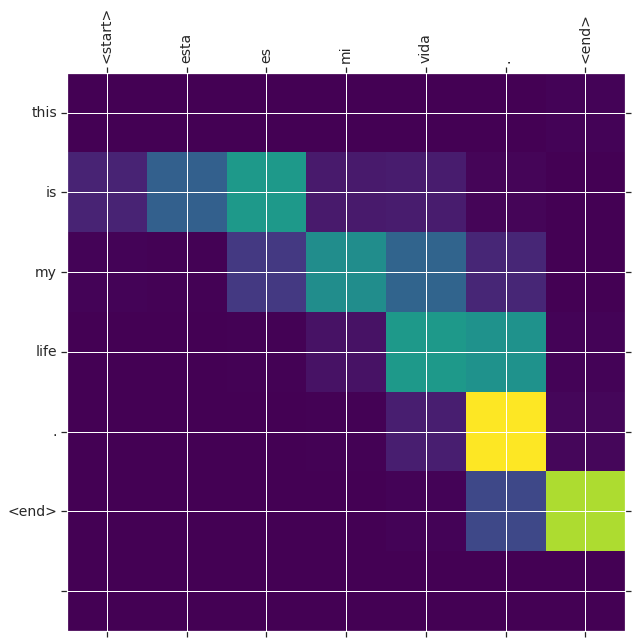（待续…）